# Placing Shapes in Order (2)

In this worksheet, students place 2D and 3D shapes in order according to the given criteria.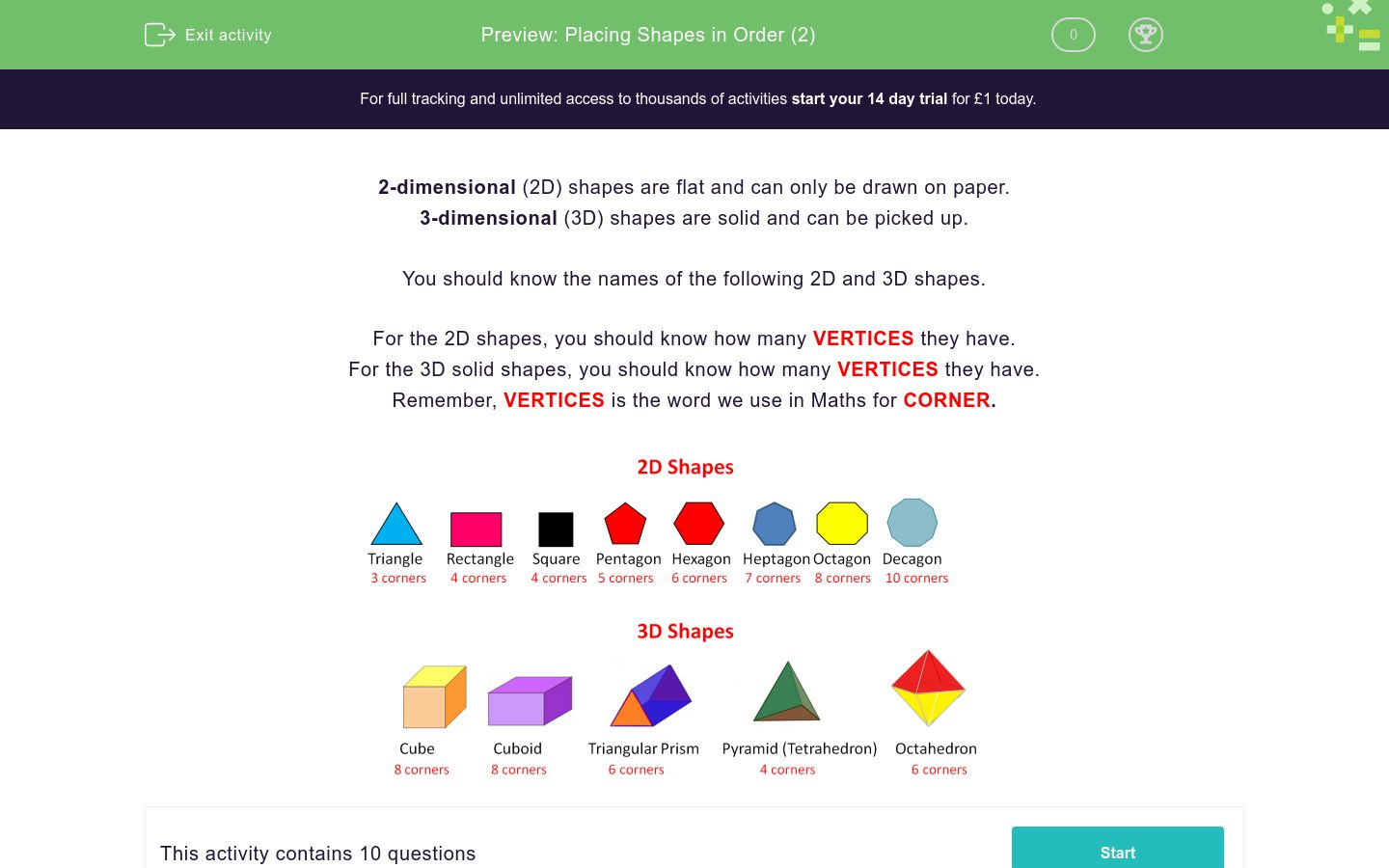Key stage:  KS 1

Curriculum topic:   Geometry: Properties of Shapes

Curriculum subtopic:   Identify 2D with 3D Shapes

Difficulty level:### QUESTION 1 of 10

2-dimensional (2D) shapes are flat and can only be drawn on paper.

3-dimensional (3D) shapes are solid and can be picked up.

You should know the names of the following 2D and 3D shapes.

For the 2D shapes, you should know how many VERTICES they have.

For the 3D solid shapes, you should know how many VERTICES they have.

Remember, VERTICES is the word we use in Maths for CORNER.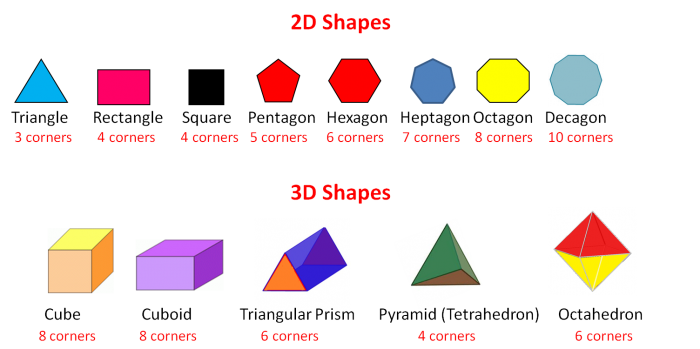Look at these 3D shapes.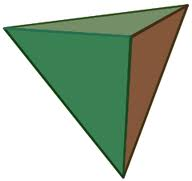Count the number of vertices on each shape.

Which shape has more vertices?

pyramid

cube

Look at these 3D shapes.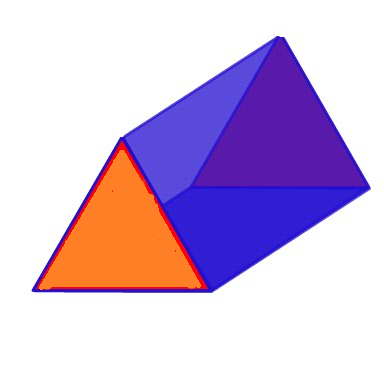Count the number of vertices on each shape.

Which shape has more vertices?

triangular prism

pyramid

Look at these 2D shapes.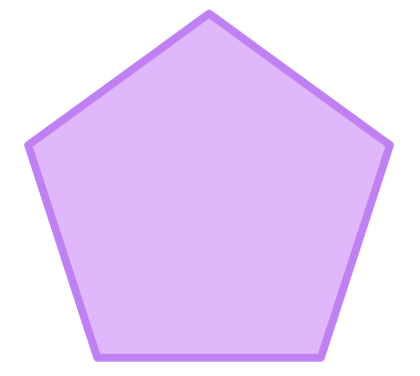Count the number of vertices on each shape and place them in order, starting with the shape that has the fewest number of vertices.

## Column B

1 (fewest)
triangle
2
square
3 (most)
pentagon

Look at these 2D shapes.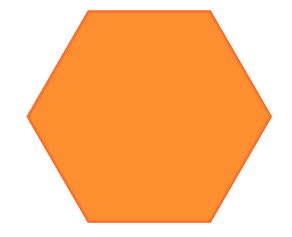Count the number of vertices on each shape and place them in order, starting with the shape that has the fewest number of vertices.

## Column B

1 (fewest)
pentagon
2
rectangle
3 (most)
hexagon

Look at these 2D shapes.Count the number of vertices on each shape and place them in order, starting with the shape that has the fewest number of vertices.

## Column B

1 (fewest)
octagon
2
hexagon
3 (most)
heptagon

Look at these 2D shapes.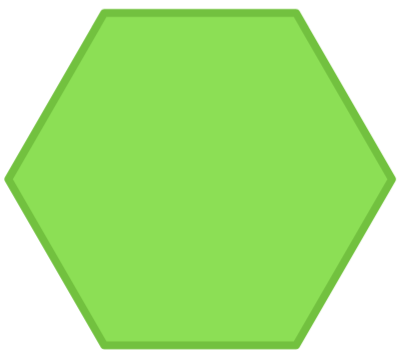Count the number of vertices on each shape and place them in order, starting with the shape that has the fewest number of vertices.

## Column B

1 (fewest)
rectangle
2
triangle
3
pentagon
4 (most)
hexagon

Look at these 2D shapes.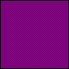Count the number of vertices on each shape and place them in order, starting with the shape that has the fewest number of vertices.

## Column B

1 (fewest)
hexagon
2
decagon
3
square
4 (most)
pentagon

Look at these 3D shapes.Count the number of vertices on each shape.

Which shape has more vertices?

triangular prism

cuboid

Look at these 3D shapes.Count the number of vertices on each shape and place them in order, starting with the shape that has the fewest number of vertices.

## Column B

1 (fewest)
pyramid
2
triangular prism
3 (most)
cuboid

Look at these 3D shapes.Count the number of vertices on each shape and place them in order, starting with the shape that has the fewest number of vertices.

## Column B

1 (fewest)
cube
2
octahedron
3 (most)
pyramid
• Question 1

Look at these 3D shapes.Count the number of vertices on each shape.

Which shape has more vertices?

cube
EDDIE SAYS
The cube has 8 vertices.
The pyramid (tetrahedron) has 4 vertices.
• Question 2

Look at these 3D shapes.Count the number of vertices on each shape.

Which shape has more vertices?

triangular prism
EDDIE SAYS
The triangular prism has 6 vertices.
The pyramid (tetrahedron) has 4 vertices.
• Question 3

Look at these 2D shapes.Count the number of vertices on each shape and place them in order, starting with the shape that has the fewest number of vertices.

## Column B

1 (fewest)
triangle
2
square
3 (most)
pentagon
EDDIE SAYS
The triangle has 3 vertices.
The square has 4 vertices.
The pentagon has 5 vertices.
• Question 4

Look at these 2D shapes.Count the number of vertices on each shape and place them in order, starting with the shape that has the fewest number of vertices.

## Column B

1 (fewest)
rectangle
2
pentagon
3 (most)
hexagon
EDDIE SAYS
The rectangle has 4 vertices.
The pentagon has 5 vertices.
The hexagon has 6 vertices.
• Question 5

Look at these 2D shapes.Count the number of vertices on each shape and place them in order, starting with the shape that has the fewest number of vertices.

## Column B

1 (fewest)
hexagon
2
heptagon
3 (most)
octagon
EDDIE SAYS
The hexagon has 6 vertices.
The heptagon has 7 vertices.
The octagon has 8 vertices.
• Question 6

Look at these 2D shapes.Count the number of vertices on each shape and place them in order, starting with the shape that has the fewest number of vertices.

## Column B

1 (fewest)
triangle
2
rectangle
3
pentagon
4 (most)
hexagon
EDDIE SAYS
The triangle has 3 vertices.
The rectangle has 4 vertices.
The pentagon has 5 vertices.
The hexagon has 6 vertices.
• Question 7

Look at these 2D shapes.Count the number of vertices on each shape and place them in order, starting with the shape that has the fewest number of vertices.

## Column B

1 (fewest)
square
2
pentagon
3
hexagon
4 (most)
decagon
EDDIE SAYS
The square has 4 vertices.
The pentagon has 5 vertices.
The hexagon has 6 vertices.
The decagon has 10 vertices.
• Question 8

Look at these 3D shapes.Count the number of vertices on each shape.

Which shape has more vertices?

cuboid
EDDIE SAYS
The triangular prism has 6 vertices.
The cuboid has 8 vertices.
• Question 9

Look at these 3D shapes.Count the number of vertices on each shape and place them in order, starting with the shape that has the fewest number of vertices.

## Column B

1 (fewest)
pyramid
2
triangular prism
3 (most)
cuboid
EDDIE SAYS
The pyramid (tetrahedron) has 4 vertices.
The triangular prism has 6 vertices.
The cuboid has 8 vertices.
• Question 10

Look at these 3D shapes.Count the number of vertices on each shape and place them in order, starting with the shape that has the fewest number of vertices.

## Column B

1 (fewest)
pyramid
2
octahedron
3 (most)
cube
EDDIE SAYS
The pyramid (tetrahedron) has 4 vertices.
The octahedron has 6 vertices.
The cube has 8 vertices.
---- OR ----

Sign up for a £1 trial so you can track and measure your child's progress on this activity.

### What is EdPlace?

We're your National Curriculum aligned online education content provider helping each child succeed in English, maths and science from year 1 to GCSE. With an EdPlace account you’ll be able to track and measure progress, helping each child achieve their best. We build confidence and attainment by personalising each child’s learning at a level that suits them.

Get started